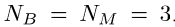# How we can write the below equation in CVX acceptable form?

Hello Everyone,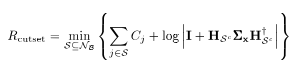Suppose you had just a single value of \mathcal{S}. How would you write the formula in MATLAB?

Hi mcg,

Considering Σx=P(power)*I(Identity) , then it would be C+log(abs( I + H * P * I * H’ ))

sorry in my previous reply, it should be determinant instead of absolute…

This can’t be right; you are trying to take the logarithm of a matrix? And which is the optimization variable?

But I’m also going to have to refer you to the following: Why isn’t CVX accepting my model? READ THIS FIRST!

Ah, I see, sorry I missed this. Still, we need to know what the optimization variable is. Certainly, log_det is concave if its argument is affine. So you could in theory maximize this quantity.

Hi mcg,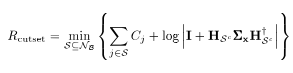Thanks for your reply. In the above equation, H is the optimization variable which is called as the channel matrix, whose elements are i.i.d. complex gaussian distributed withwhere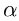is the cross channel gain, i ∈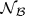and k ∈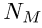where